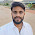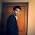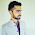# Wish Christmas with SAS

This post is dedicated to the lovers of SAS programming language. Flaunt your knowledge in your peer group with the following SAS program 😊 It's one of the stupidest and coolest way to make simple things complex. The idea is to show off your creativity and solve easy problems with complicated ways to learn some cool tricks which can be used to solve future real complex problems.Be Creative with SAS
Run the following program and see what i mean -
DATA A(KEEP=WISH);
LENGTH WISH \$15 F1 \$5 F2 \$9;
DO J= 1 TO 5;
IF J = 1 THEN K = TAN(4.702389315) / ATAN(3.584020431);
ELSE IF J = 2 THEN K = SUM(INPUT(PUT('99',\$HEX2.),8.),             INPUT(PUT('0',\$HEX2.),8.));
ELSE IF J <= 4 THEN K = SUBSTR(PUT('12JAN2004'D , 5.),4, 2);
ELSE IF J = 5 THEN K = ROUND(CONSTANT("PI")*30, 10) - 1;
SUBSTR(F1,J)=BYTE(K);
END;

DO I= 1 TO 9;
IF I = 1 THEN K = 100 / ARCOS(0.078190328);
ELSE IF I = 2 THEN K = MOD(CONSTANT("PI") / 3, 2*CONSTANT("PI")) * 360/(2*CONSTANT("PI")) + 3*4;
ELSE IF I = 3 THEN K = SUBSTR(PUT('12JAN2004'D , 5.),4, 2);
ELSE IF I = 4 THEN K = ROUND(2**6.189823562,1);
ELSE IF I = 5 THEN K = EXP(4.418841708);
ELSE IF I = 6 THEN K = MEDIAN(82,86);
ELSE IF I = 7 THEN K = TAN(4.702389315) / ATAN(3.584020431);
ELSE IF I = 8 THEN K = SUM(INPUT(PUT('69',\$HEX2.),8.)-1, INPUT(PUT('0',\$HEX2.),8.));
ELSE IF I = 9 THEN K = EXP(4.418841708);
SUBSTR(F2,I)=BYTE(K);
END;
WISH = CATX(' ',F1,F2);
OUTPUT;
PROC PRINT NOOBS;
RUN;

Output

The output of the program is not shown here for surprises. Please post your output in the comment box below!

How it works

1. You can create character value with BYTE() function.  The BYTE function returns character value against the specified ASCII code. Run BYTE(65) and see what it evaluates. Run the program below and see log.
data _null_;
x = byte(65);
put x=;
run;
Result : A . The above program returns A.

Similarly, you can create A to Z alphabets with the following program.
data _null_;
do i = 65 to 90;
x = byte(i);
put x;
end;
run;
See log after submitting the above code.

2. What Trigonometric function does in the code -

Step 1 : TAN(4.702389315) returns 100
Step 2 : ATAN(3.584020431) returns 1.2987
Step 3 : = 100 / 1.2987 is equal to 77
Step 4:  BYTE(77) returns M of 'MERRY'

3. How to know exact angle before using in TRIGNO functions

Use Goal Seek Feature of MS Excel. See the GOAL SEEK TutorialRelated PostsDeepanshu founded ListenData with a simple objective - Make analytics easy to understand and follow. He has over 8 years of experience in data science. During his tenure, he has worked with global clients in various domains like Banking, Insurance, Telecom and Human Resource.

6 Responses to "Wish Christmas with SAS"
1.WISH
MERRY CHRISTMAS

2.WISH
MERRY CHRISTMAS

3.Hello all!.....
Can i have the output for "Print out the middlest observation in a dataset"?

1.In the code below, "mydata" is your dataframe name.

data test;
if 0 then set mydata nobs=n;
n = ceil(n/2);
set mydata point=n;
output;
stop;
proc print;
run;

4.Codes for Happy New Year 2019 in SAS

5.Hey friend, it is very well written article, thank you for the valuable and useful information you provide in this post. Keep up the good work! FYI, please check these depression, stress and anxiety related articles:

Happy New Year Wallpaper, Santa Claus Wallpaper,
Latest Christmas Wallpaper, Jesus Christ Wallpaper,